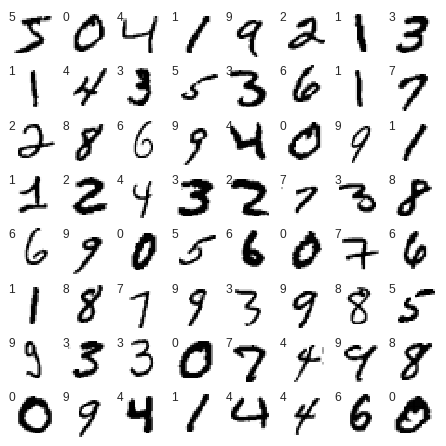1. Load the digit dataset¶

1.1 Spliting data into train and test sets¶

In :
(X_train, y_train), (X_test, y_test) = mnist.load_data()
11493376/11490434 [==============================] - 0s 0us/step

1.2 Checking the shape of the input data¶

In :
print('x_train:\t{}' .format(X_train.shape))
print('y_train:\t{}' .format(y_train.shape))
print('x_test:\t\t{}'.format(X_test.shape))
print('y_test:\t\t{}'.format(y_test.shape))
x_train:	(60000, 28, 28)
y_train:	(60000,)
x_test:		(10000, 28, 28)
y_test:		(10000,)

1.3 Plot first 64 digits¶

Now we can plot some digits to see how they look.

digits.target contain real value for each image in digits.images

In :
# set up the figure
fig = plt.figure(figsize=(6, 6))
fig.subplots_adjust(left=0, right=1, bottom=0, top=1, hspace=0.05, wspace=0.05)

# plot the digits: each image is 28x28 pixels
for i in range(64):
ax = fig.add_subplot(8, 8, i + 1, xticks=[], yticks=[])
ax.imshow(X_train[i], cmap=plt.cm.binary, interpolation='nearest')

# label the image with the target value
ax.text(0, 7, str(y_train[i]))In :
img_rows, img_cols = 28, 28

X_train = X_train.reshape(X_train.shape, img_rows, img_cols, 1)
X_test = X_test.reshape(X_test.shape, img_rows, img_cols, 1)

X_train = X_train.astype('float32')
X_test = X_test.astype('float32')

X_train /= 255.
X_test /= 255.

print('X_train:\t{}' .format(X_train.shape),' -reshaped')
print('X_test: \t{}' .format(X_test.shape),' -reshaped')
X_train:	(60000, 28, 28, 1)  -reshaped
X_test: 	(10000, 28, 28, 1)  -reshaped

1.4 One Hot Encoding¶

Many machine learning algorithms cannot operate on label data directly. They require all input variables and output variables to be numeric.

This means that categorical data must be converted to a numerical form.

In :
# one hot encode outputs
y_train = np_utils.to_categorical(y_train)
y_test = np_utils.to_categorical(y_test)
num_classes = y_test.shape